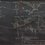# A binomial sum

It's not too hard to show that $$\displaystyle \binom{-\frac{1}{2}}{n} = \left( -\dfrac{1}{4} \right)^n \binom{2n}{n}$$ and hence, $$\displaystyle \frac{1}{\sqrt{1-4x}} = \sum_{n=0}^\infty \binom{-\frac{1}{2}}{n}(-4x)^n = \sum_{n=0}^\infty \binom{2n}{n}x^n$$.

Some tinkering around on WolframAlpha revealed that $\displaystyle \sum_{n=0}^\infty \binom{3n}{n}x^n = \frac{2\cos(\frac{1}{3}\sin^{-1}(\frac{3\sqrt{3}}{2}\sqrt{x}))}{\sqrt{4-27x}}$. By a rather natural substitution $x = \frac{4}{27} \sin^2 \theta$, we get

$\sum_{n=0}^\infty \binom{3n}{n}x^n = \boxed{\dfrac{\cos \frac{\theta}{3}}{\cos \theta}}.$

Sadly, WA revealed nothing about $\displaystyle \sum_{n=0}^\infty \binom{4n}{n}x^n$. A cool expression for $\displaystyle \sum_{n=0}^\infty \binom{4n}{2n}x^n$ is easily obtained with the substitution $x = \frac{1}{16}\sin^2 \theta$, however:

\begin{aligned} \sum_{n=0}^\infty \binom{4n}{2n}x^n &= \frac{1}{2} \left[ \sum_{n=0}^\infty \binom{2n}{n}(\sqrt{x})^n + \sum_{n=0}^\infty \binom{2n}{n}(-\sqrt{x})^n \right] \\ &= \frac{1}{2} \left[ \frac{1}{\sqrt{1-4\sqrt{x}}} + \frac{1}{\sqrt{1+4\sqrt{x}}} \right] \\ &= \frac{1}{2} \left[ \frac{\sqrt{1-4\sqrt{x}}+\sqrt{1+4\sqrt{x}}}{\sqrt{1-16x}} \right] \\ &= \frac{1}{2} \left[ \frac{\sqrt{1-\sin \theta}+\sqrt{1+\sin \theta}}{\cos \theta} \right] \\ &= \frac{1}{2} \left[ \frac{\sqrt{2 + 2\cos \theta}}{\cos \theta} \right] \\ &= \boxed{\dfrac{\cos \frac{\theta}{2}}{\cos \theta}}. \end{aligned}

Let's define the sum $\displaystyle F_{a,b}(x) = \sum_{n=0}^\infty \binom{an}{bn}x^n$. For convenience let's also define $K_{a,b} = \dfrac{b^b(a-b)^{a-b}}{a^a}$. So $K_{2,1} = \dfrac{1}{4}$, $K_{3,1} = \dfrac{4}{27}$, and $K_{4,2} = \dfrac{1}{16}$. It seems that the factor associated with the $\sin^2 \theta$ comes from the series' radius of convergence, ie

\begin{aligned} \binom{an}{bn} &= \frac{(an)!}{(bn)!((a-b)n)!} \\ &\sim \frac{\text{Insert Stirling's approximation}}{\text{Insert more Stirling's approximations}} \\ &\sim Cn^{-1/2} \left( \frac{a^a}{b^b(a-b)^{a-b}} \right)^n \\ &\sim Cn^{-1/2} K_{a,b}^{-n}. \end{aligned}

Conjecture. $\displaystyle F_{a,b}(K_{a,b}\sin^2 \theta) = \frac{\text{Some expression}}{\cos \theta}$.Note by Jake Lai
2 years, 11 months ago

This discussion board is a place to discuss our Daily Challenges and the math and science related to those challenges. Explanations are more than just a solution — they should explain the steps and thinking strategies that you used to obtain the solution. Comments should further the discussion of math and science.

When posting on Brilliant:

• Use the emojis to react to an explanation, whether you're congratulating a job well done , or just really confused .
• Ask specific questions about the challenge or the steps in somebody's explanation. Well-posed questions can add a lot to the discussion, but posting "I don't understand!" doesn't help anyone.
• Try to contribute something new to the discussion, whether it is an extension, generalization or other idea related to the challenge.

MarkdownAppears as
*italics* or _italics_ italics
**bold** or __bold__ bold
- bulleted- list
• bulleted
• list
1. numbered2. list
1. numbered
2. list
Note: you must add a full line of space before and after lists for them to show up correctly
paragraph 1paragraph 2

paragraph 1

paragraph 2

[example link](https://brilliant.org)example link
> This is a quote
This is a quote
    # I indented these lines
# 4 spaces, and now they show
# up as a code block.

print "hello world"
# I indented these lines
# 4 spaces, and now they show
# up as a code block.

print "hello world"
MathAppears as
Remember to wrap math in $$ ... $$ or $ ... $ to ensure proper formatting.
2 \times 3 $2 \times 3$
2^{34} $2^{34}$
a_{i-1} $a_{i-1}$
\frac{2}{3} $\frac{2}{3}$
\sqrt{2} $\sqrt{2}$
\sum_{i=1}^3 $\sum_{i=1}^3$
\sin \theta $\sin \theta$
\boxed{123} $\boxed{123}$